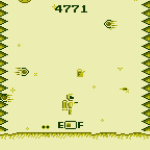-=+=- -=+=- -=+=- -=+=- -=+=- -=+=- -=+=- -=+=- -=+=- -=+=- -=+=- -=+=- -=+=- -=+=- -=+=- -=+=- -=+=- -=+=- -=+=- -=+=- -=+=- -=+=- -=+=- -=+=- -=+=- -=+=- -=+=- -=+=- -=+=- -=+=- (c) WidthPadding Industries 1987 0|489|0 -=+=- -=+=- -=+=- -=+=- -=+=- -=+=- -=+=- -=+=- -=+=- -=+=- -=+=- -=+=- -=+=- -=+=- -=+=- -=+=- -=+=- -=+=- -=+=- -=+=- -=+=- -=+=- -=+=- -=+=- -=+=- -=+=- -=+=- -=+=- -=+=- -=+=-
SoCoder -> Showcase Home -> Retro

rychanCreated : 06 January 2016

System : Other
Language : C/C++

Novascape

A fun Gameboy ROM in 48 hours for LD 34

Gameboy ROM
ScreenshotsNovascape is a game that ended up as a cross between river raid and one of the many spaceman thrust games of the early-mid 80's. Featuring 2 difficulty settings, some fairly tough gameplay, nice sound effects and ending sequence!

All in under 64kb!A lovely game. Nice and hard, just the way a game oughta beI do enjoy a challenge apparently, guess it comes across in the games I make